# Titration Volumetric analysis Titration Volumetric analysis relies on

• Slides: 31Titration Volumetric analysisTitration Ø Volumetric analysis relies on methods involving the accurate measurement of volumes of solutions, although mass measurements may also be required. Ø Essentially, we measure the volume of a standard solution (one of accurately known concentration) needed to react exactly with a known volume of another solution (one of unknown concentration) in a chemical reaction for which the stoichiometric or balanced chemical equation is known. Ø From the data, we are then in a position to calculate the accurate concentration of the second solution. Ø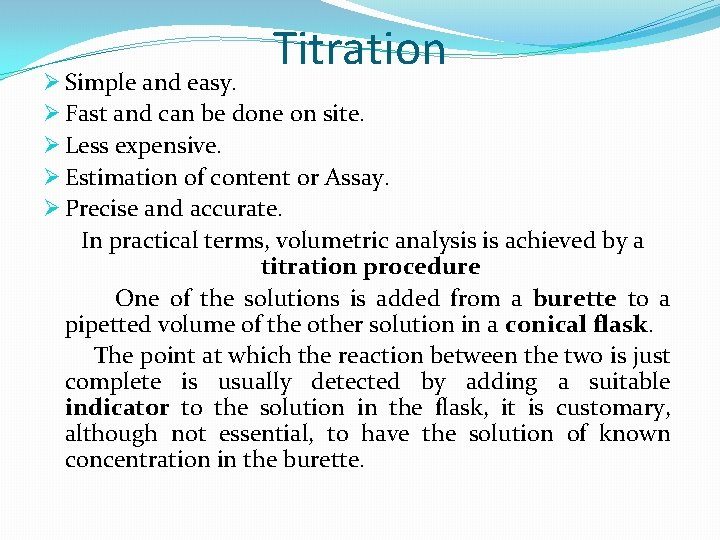Titration Ø Simple and easy. Ø Fast and can be done on site. Ø Less expensive. Ø Estimation of content or Assay. Ø Precise and accurate. In practical terms, volumetric analysis is achieved by a titration procedure One of the solutions is added from a burette to a pipetted volume of the other solution in a conical flask. The point at which the reaction between the two is just complete is usually detected by adding a suitable indicator to the solution in the flask, it is customary, although not essential, to have the solution of known concentration in the burette.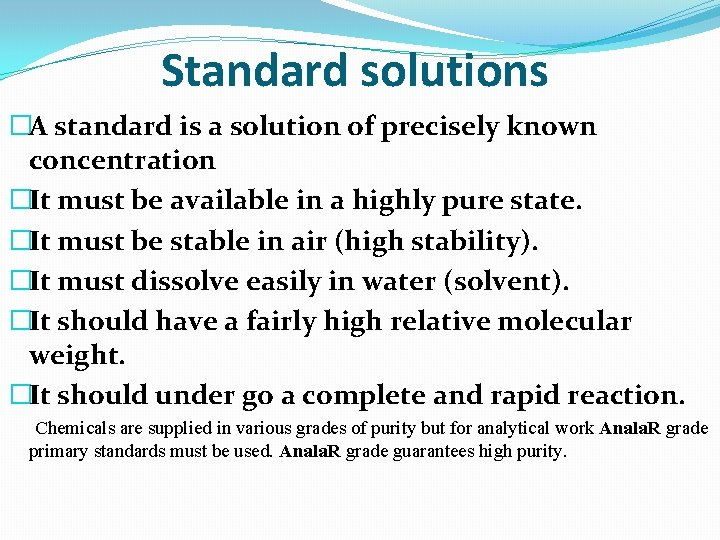Standard solutions �A standard is a solution of precisely known concentration �It must be available in a highly pure state. �It must be stable in air (high stability). �It must dissolve easily in water (solvent). �It should have a fairly high relative molecular weight. �It should under go a complete and rapid reaction. Chemicals are supplied in various grades of purity but for analytical work Anala. R grade primary standards must be used. Anala. R grade guarantees high purity.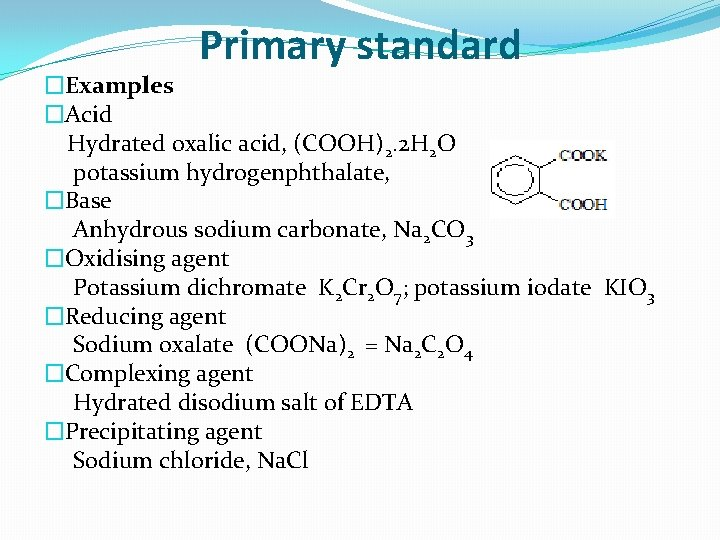Primary standard �Examples �Acid Hydrated oxalic acid, (COOH)2. 2 H 2 O potassium hydrogenphthalate, �Base Anhydrous sodium carbonate, Na 2 CO 3 �Oxidising agent Potassium dichromate K 2 Cr 2 O 7; potassium iodate KIO 3 �Reducing agent Sodium oxalate (COONa)2 = Na 2 C 2 O 4 �Complexing agent Hydrated disodium salt of EDTA �Precipitating agent Sodium chloride, Na. Cl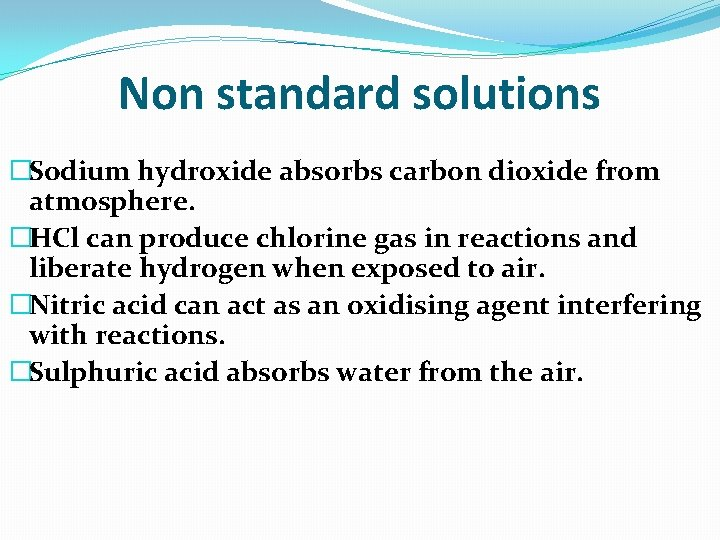Non standard solutions �Sodium hydroxide absorbs carbon dioxide from atmosphere. �HCl can produce chlorine gas in reactions and liberate hydrogen when exposed to air. �Nitric acid can act as an oxidising agent interfering with reactions. �Sulphuric acid absorbs water from the air.Calculation of Results from titration The calculation of the titration depend on chemical equation balanced, to determine the concentration of unknown solution by standard solution, (the equivalents No. of unknown equal to the equivalents No. of standard), standard for general reaction: a. A+b. B c. C+d. D no. Eq. of A = no. Eq. of B no. Eq. = N x V = Wt. / Eq. Wt. NA x V A = N B x V B (V A MA/a) = (VB MB/b) Eq. Wt. of B = M. Wt. / a Wt. of B = NA x VA x Eq. Wt. of B Wt% of B = (Wt. of B / Wt. of sample ) x 100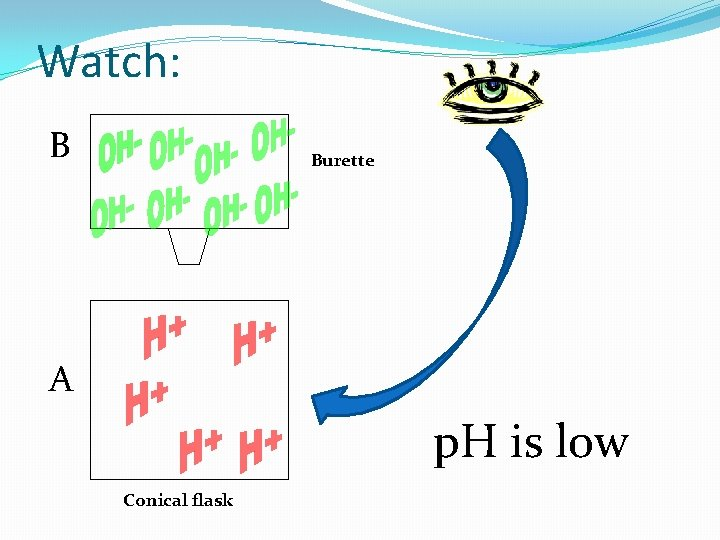Watch: B Burette A p. H is low Conical flaskWatch: B A p. H is getting higherWatch: B A p. H is even higherWatch: B A p. H is even higher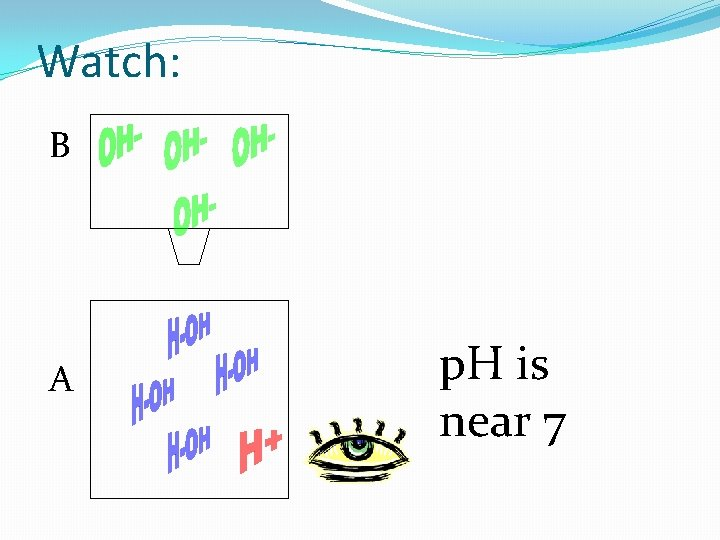Watch: B A p. H is near 7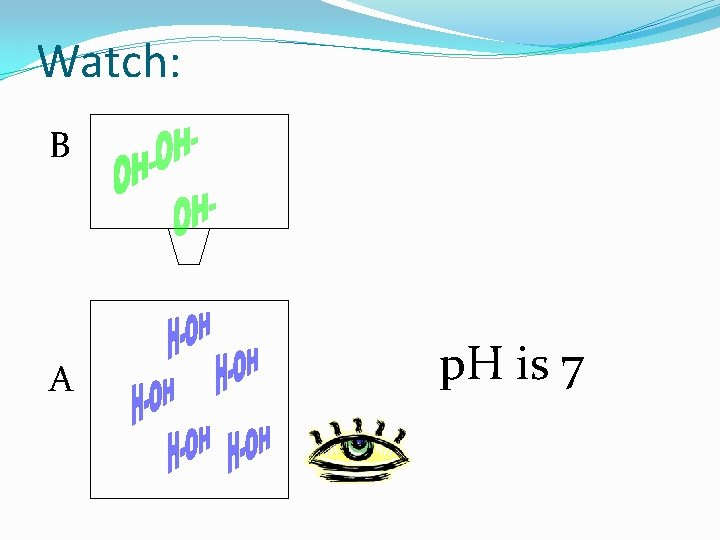Watch: B A p. H is 7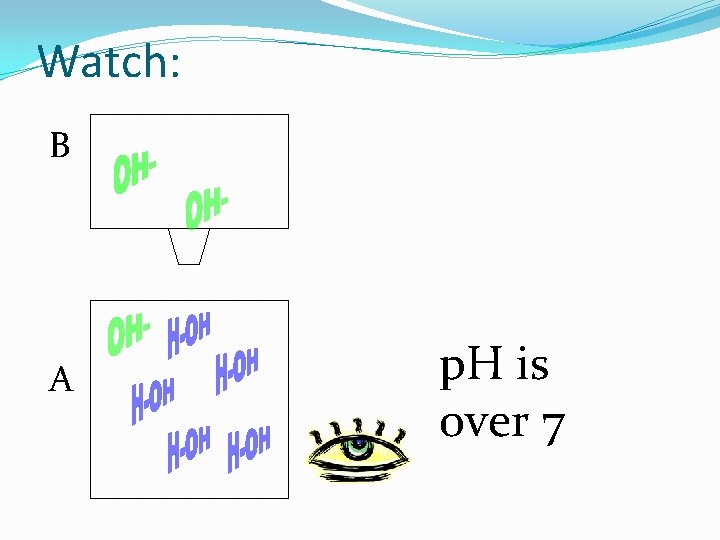Watch: B A p. H is over 7Titration of Sodium Carbonate with Hydrochloric acid Na 2 CO 3 + 2 HCl 2 Na. Cl + H 2 O + CO 2 1 mole 2 moles 1 moleApparatus used �Burette. �Conical flask. �Beaker. �Pipette. �Funnel. �White tile.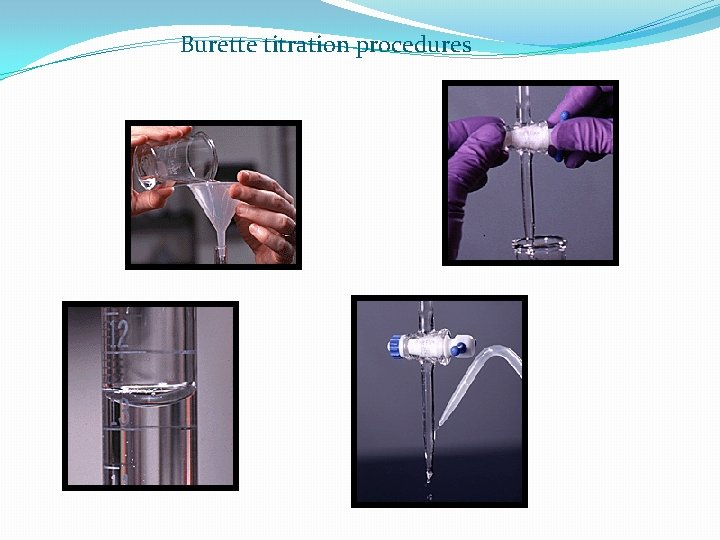Burette titration procedures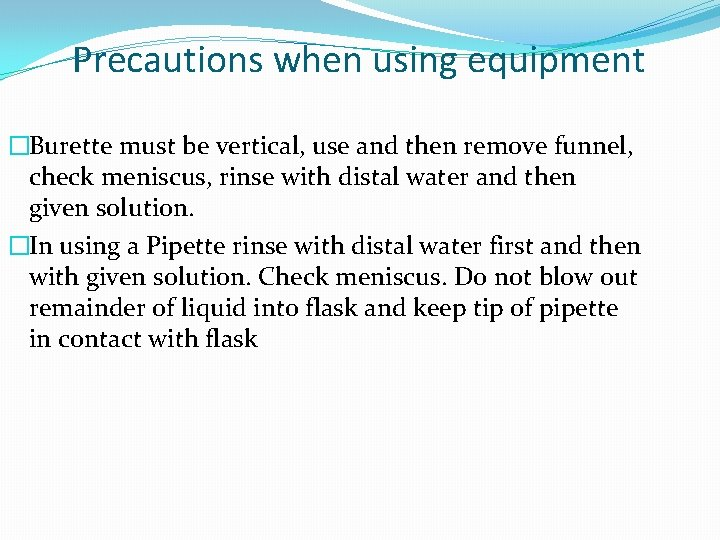Precautions when using equipment �Burette must be vertical, use and then remove funnel, check meniscus, rinse with distal water and then given solution. �In using a Pipette rinse with distal water first and then with given solution. Check meniscus. Do not blow out remainder of liquid into flask and keep tip of pipette in contact with flaskPrecautions �Conical flask should not be rinsed with solution it is to contain and swirl �In using a Volumetric flask the last few m. L must be added so that the meniscus rest on calibration mark �Invert stoppered flask to ensure solution is homogeneous/uniform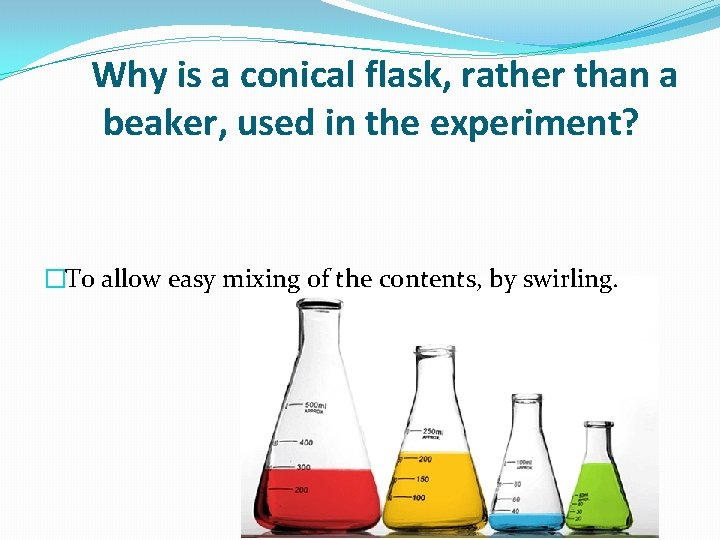Why is a conical flask, rather than a beaker, used in the experiment? �To allow easy mixing of the contents, by swirling.Why is the funnel removed from the burette after adding the acid solution? �So that drops of solution from the funnel will not fall into the burette.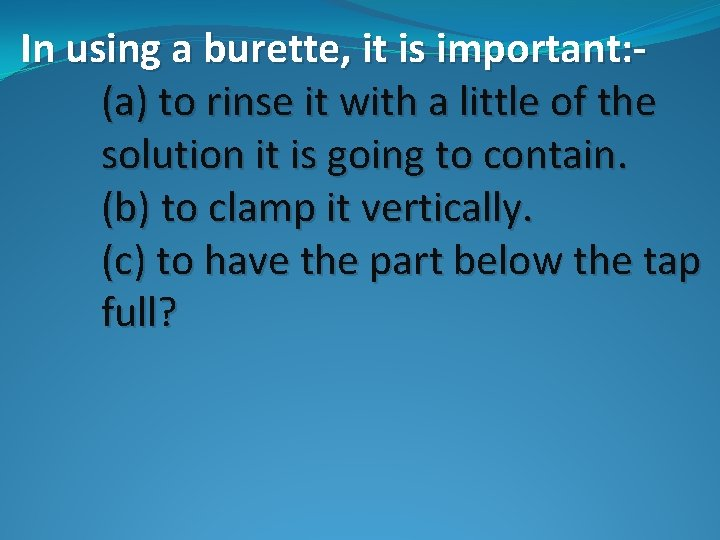In using a burette, it is important: (a) to rinse it with a little of the solution it is going to contain. (b) to clamp it vertically. (c) to have the part below the tap full?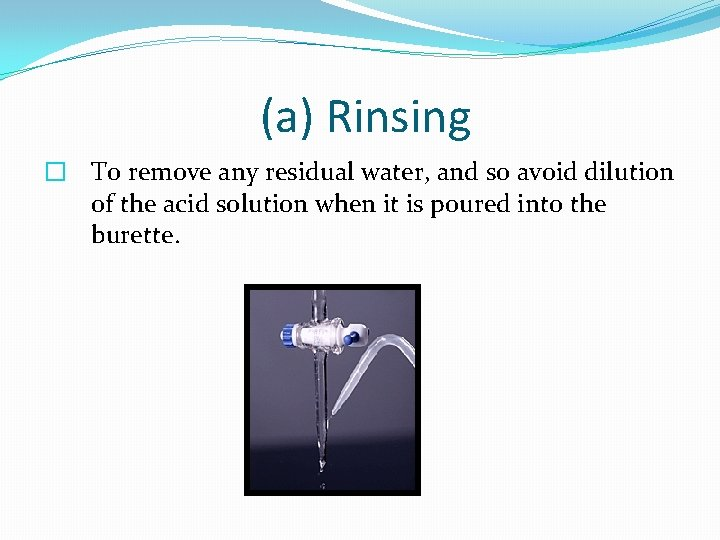(a) Rinsing � To remove any residual water, and so avoid dilution of the acid solution when it is poured into the burette.(b) clamp vertically �To enable the liquid level to be read correctly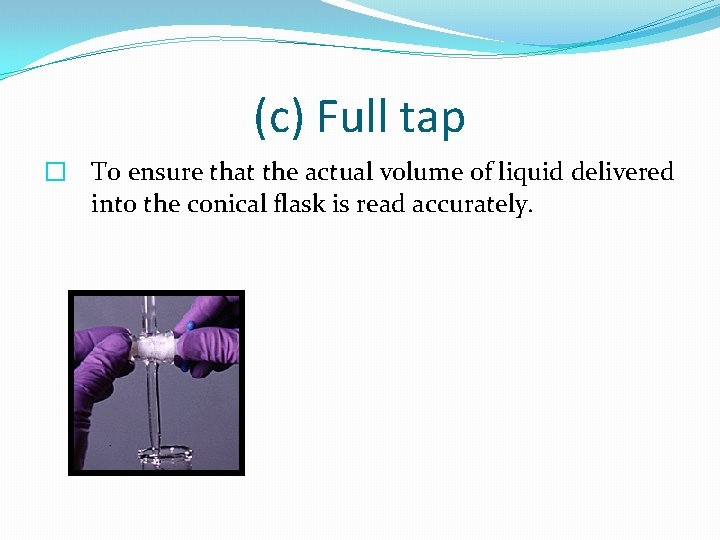(c) Full tap � To ensure that the actual volume of liquid delivered into the conical flask is read accurately.The following procedures were carried out during the titration: The sides of the conical flask were washed down with distal water. The conical flask was frequently swirled or shaken. Give one reason for carrying out each of these procedures.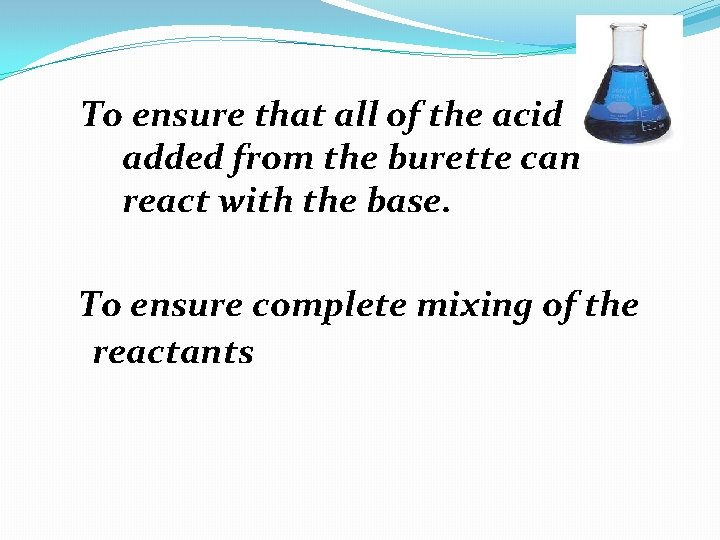To ensure that all of the acid added from the burette can react with the base. To ensure complete mixing of the reactants� Why is a rough titration carried out? � To find the approximate end-point. This information enables the subsequent titrations to be carried out more quickly. � Why is more than one accurate titration carried out? � To minimise error by getting accurate readings within 0. 1 m. L of each other.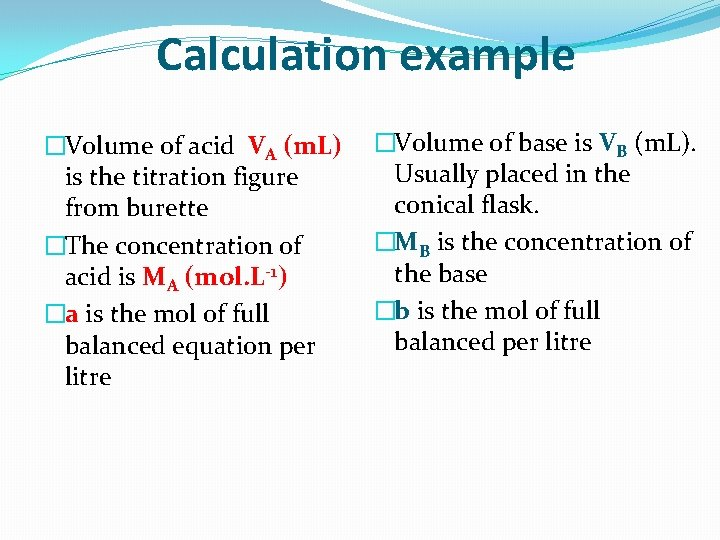Calculation example �Volume of acid VA (m. L) is the titration figure from burette �The concentration of acid is MA (mol. L-1) �a is the mol of full balanced equation per litre �Volume of base is VB (m. L). Usually placed in the conical flask. �MB is the concentration of the base �b is the mol of full balanced per litre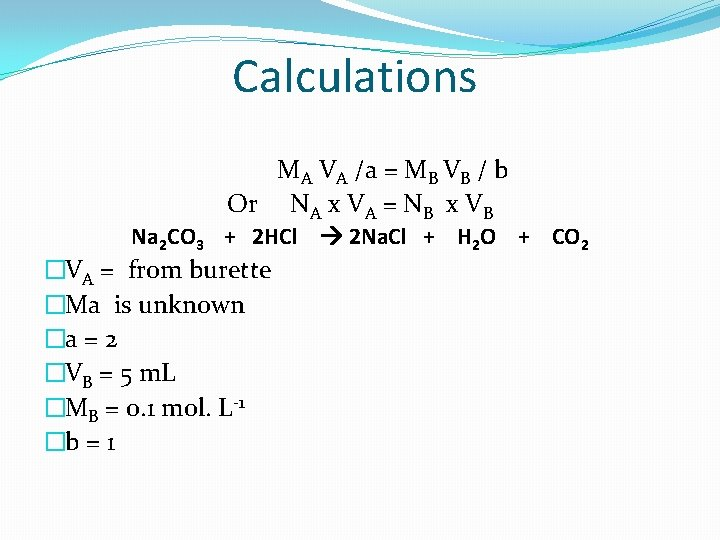Calculations MA VA /a = MB VB / b Or NA x VA = NB x VB Na 2 CO 3 + 2 HCl 2 Na. Cl + H 2 O + CO 2 �VA = from burette �Ma is unknown �a = 2 �VB = 5 m. L �MB = 0. 1 mol. L-1 �b = 1THE END